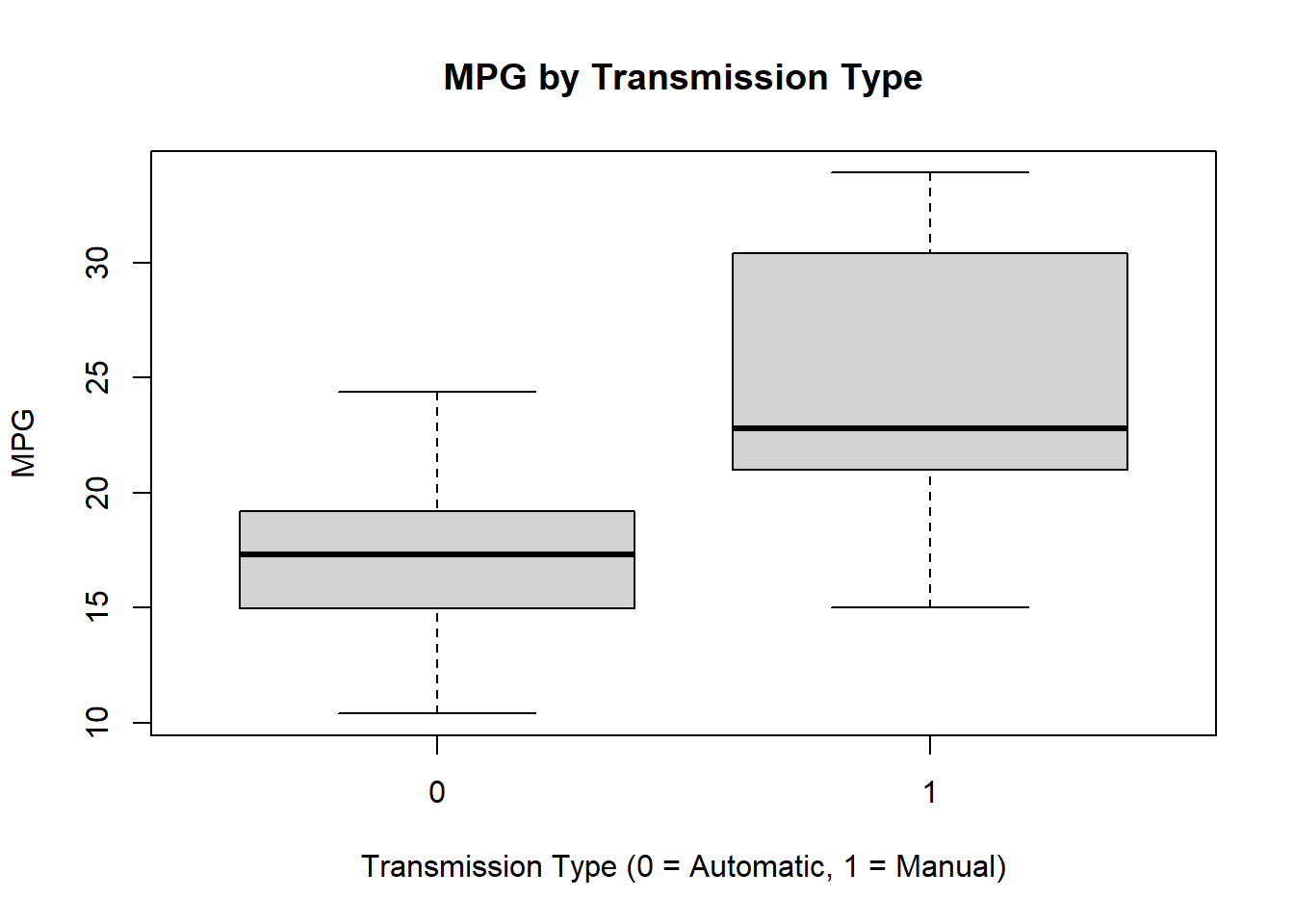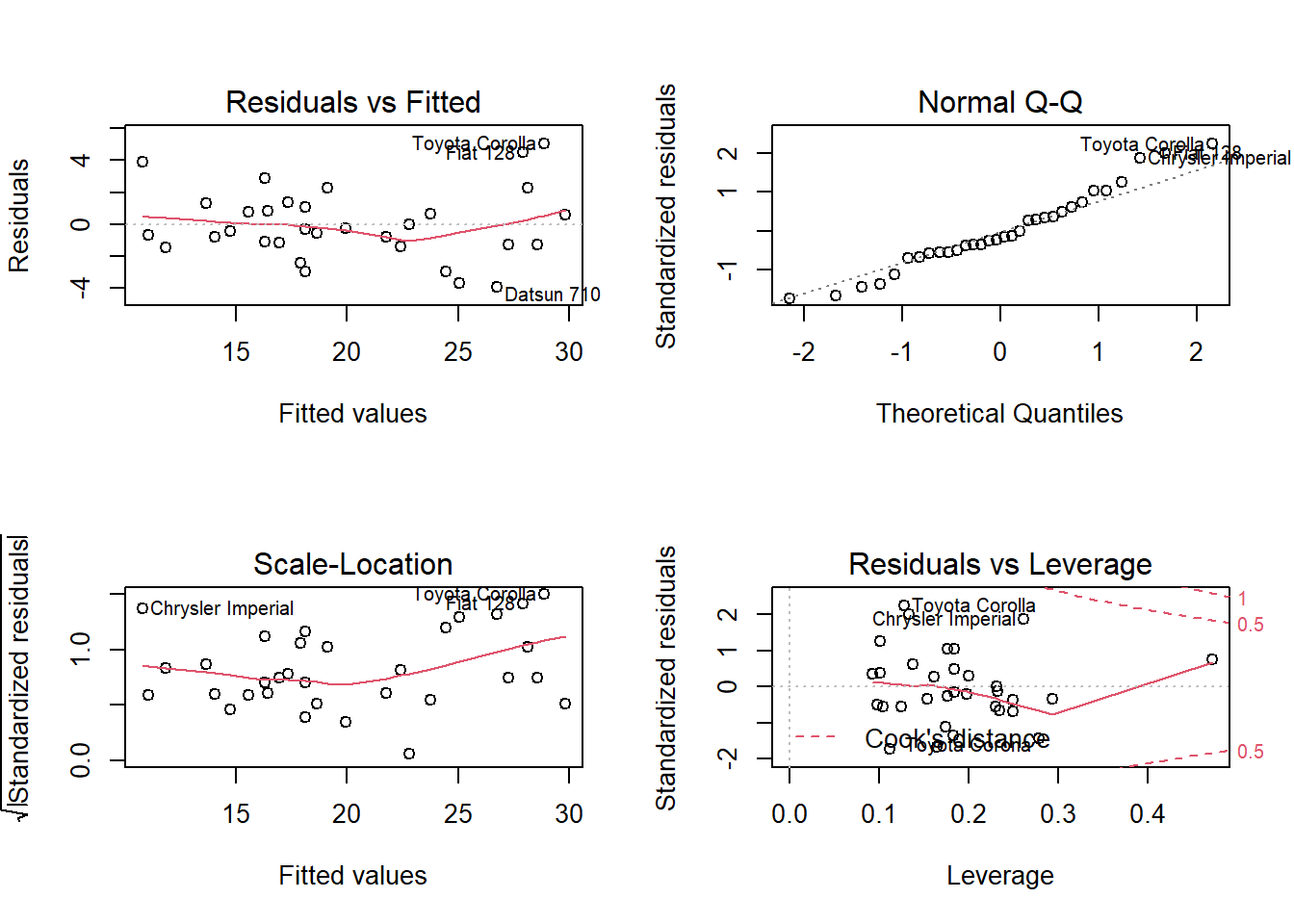## R Markdown

Created with knitr

#### Executive Summary

This report analyzed the relationship between transmission type (manual or automatic) and miles per gallon (MPG). The report set out to determine which transmission type produces a higher MPG. The mtcars dataset was used for this analysis. A t-test between automatic and manual transmission vehicles shows that manual transmission vehicles have a 7.245 greater MPG than automatic transmission vehicles. After fitting multiple linear regressions, analysis showed that the manual transmission contributed less significantly to MPG, only an improvement of 1.81 MPG. Other variables, weight, horsepower, and number of cylinders contributed more significantly to the overall MPG of vehicles.

Load the dataset and convert categorical variables to factors.

library(ggplot2)
## Warning: package 'ggplot2' was built under R version 4.1.1
data(mtcars)
dim(mtcars)
mtcars$cyl <- as.factor(mtcars$cyl)
mtcars$vs <- as.factor(mtcars$vs)
mtcars$am <- factor(mtcars$am)
mtcars$gear <- factor(mtcars$gear)
mtcars$carb <- factor(mtcars$carb)
attach(mtcars)

#### Exploratory Analysis

See Appendix Figure I Exploratory Box graph that compares Automatic and Manual transmission MPG. The graph leads us to believe that there is a significant increase in MPG when for vehicles with a manual transmission vs automatic.

##### Statistical Inference

T-Test transmission type and MPG

testResults <- t.test(mpg ~ am)
testResults$p.value ##  0.001373638 The T-Test rejects the null hypothesis that the difference between transmission types is 0. testResults$estimate
## mean in group 0 mean in group 1
##        17.14737        24.39231

The difference estimate between the 2 transmissions is 7.24494 MPG in favor of manual.

##### Regression Analysis

Fit the full model of the data

fullModelFit <- lm(mpg ~ ., data = mtcars)
summary(fullModelFit)  # results hidden
summary(fullModelFit)$coeff # results hidden Since none of the coefficients have a p-value less than 0.05 we cannot conclude which variables are more statistically significant. Backward selection to determine which variables are most statistically significant stepFit <- step(fullModelFit) summary(stepFit) # results hidden summary(stepFit)$coeff # results hidden

The new model has 4 variables (cylinders, horsepower, weight, transmission). The R-squared value of 0.8659 confirms that this model explains about 87% of the variance in MPG. The p-values also are statistically significantly because they have a p-value less than 0.05. The coefficients conclude that increasing the number of cylinders from 4 to 6 with decrease the MPG by 3.03. Further increasing the cylinders to 8 with decrease the MPG by 2.16. Increasing the horsepower is decreases MPG 3.21 for every 100 horsepower. Weight decreases the MPG by 2.5 for each 1000 lbs increase. A Manual transmission improves the MPG by 1.81.

#### Residuals & Diagnostics

Residual Plot See Appendix Figure II

The plots conclude:

1. The randomness of the Residuals vs. Fitted plot supports the assumption of independence
2. The points of the Normal Q-Q plot following closely to the line conclude that the distribution of residuals is normal
3. The Scale-Location plot random distribution confirms the constant variance assumption
4. Since all points are within the 0.05 lines, the Residuals vs. Leverage concludes that there are no outliers
sum((abs(dfbetas(stepFit)))>1)
##  0

#### Conclusion

There is a difference in MPG based on transmission type. A manual transmission will have a slight MPG boost. However, it seems that weight, horsepower, & number of cylinders are more statistically significant when determining MPG.

### Appendix Figures

#### I#### II